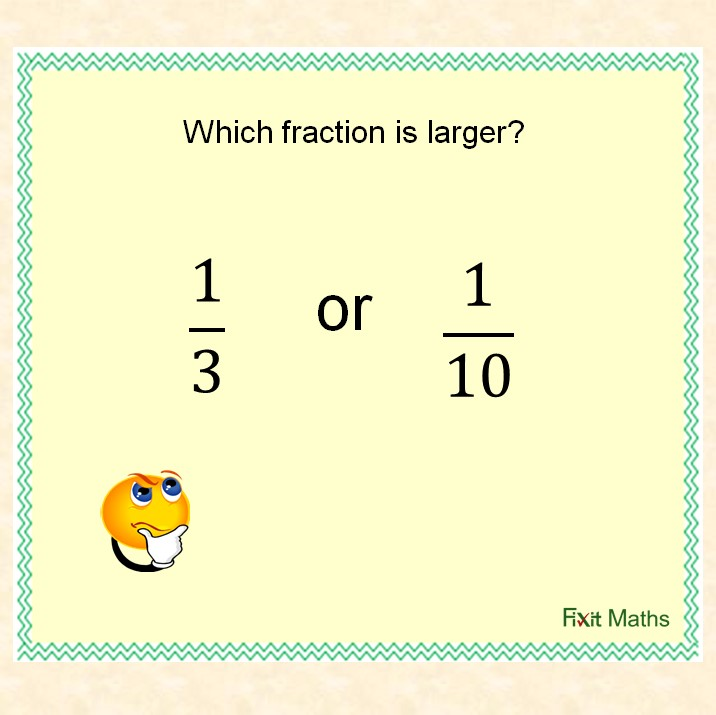## Comparing fractions

When comparing fractions, we must consider both the numerator and denominator. If either are the same, we can compare the other.If neither are the same, we must first make the fractions equivalent by finding a common denominator, to be able to compare like parts.

Understanding fractions as division can be useful when considering the relative size of fractions to compare them.